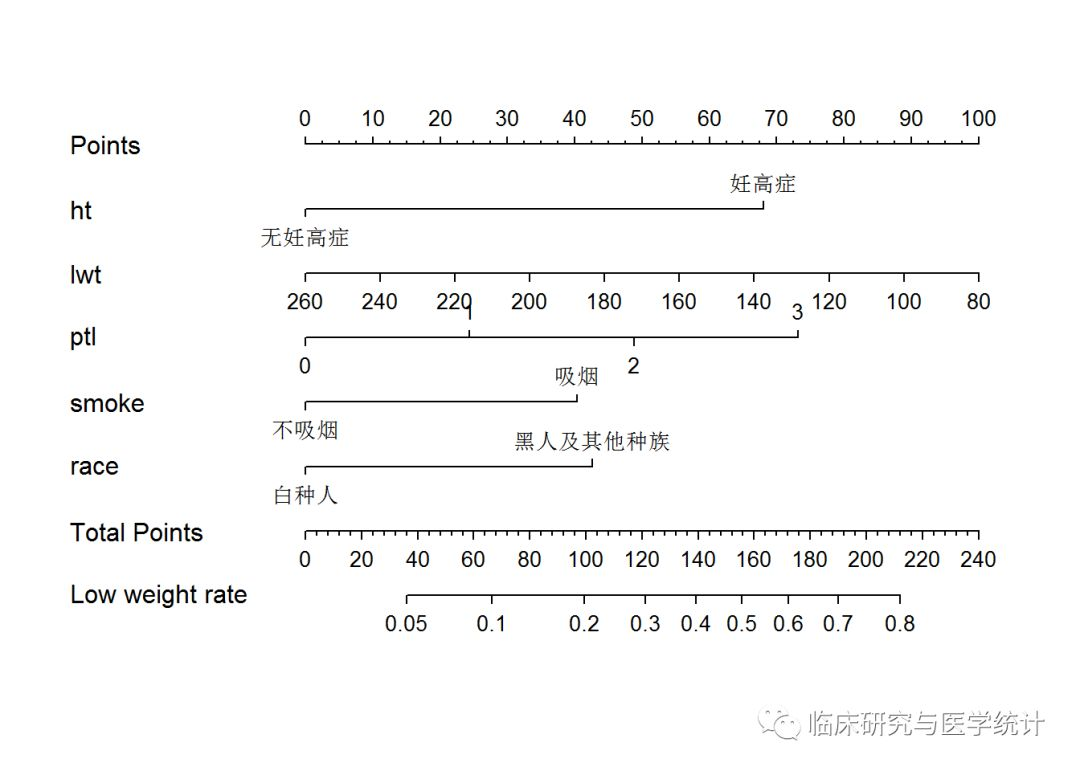# 基于R语言的Logistic回归模型构建与Nomogram绘制

1. 背景知识

（1）明确临床问题，确定科学假说

（2）根据既往文献，确定预测模型研究思路

（3）确定预测模型的预测变量

（4）确定预测模型的结局变量

（5）构建预测模型，计算模型预测值

（6）模型区分能力评估

（7）模型的准确性评估2. 案例分析

Hosmer和 Lemeshow于1989年研究了低出生体重婴儿的影响因素。结果变量为：是否娩出低出生体重儿（变量名为“low”，二分类变量，1=低出生体重，即婴儿出生体重<2500g;0=非低出生体重），考虑的影响因素（自变量）有：产妇妊娠前体重（lwt，磅）；产妇年龄（age，岁）；产妇在妊娠期间是否吸烟（smoke，0＝未吸、1＝吸烟）；本次妊娠前早产次数（ptl，次）；是否患有高血压（ht，0＝未患、1＝患病）；子宫对按摩、催产素等刺激引起收缩的应激性（ui，0＝无、1＝有）；妊娠前三个月社区医生随访次数（ftv，次）；种族（race，1＝白人、2＝黑人、3＝其他民族）。本案例因变量是二分类变量（是否低出生体重儿），研究目的是探讨低出生体重儿的独立影响因素，符合二元Logistic回归的应用条件。因为本例中，我们只有这一个数据集，可以用这个数据集作为训练集建模，然后在本数据集利用Bootstrap重抽样的方法进行模型验证。下面我们就基于R语言演示预测低出生体重儿的预测模型构建与Nomogram的绘制，我们把数据sav的数据格式整理好，命名为“lweight.sav”，保存在R语言当前工作路径下。具体分析步骤如下：

（1）首先筛选影响低出生体重儿的独立影响因素，构建Logistic回归模型；

（2）绘制Nomogram；

（3）计算模型的区分度 C-Statistics；

（4）重抽样的方法进行模型验证，并绘制Calibration曲线。

3.代码及结果解读

```library(foreign)
##
##     format.pval, units## Loading required package: SparseM## Attaching package: 'SparseM'## The following object is masked from 'package:base':
##
##     backsolve```

```mydata<-read.spss("lweight.sav")
mydata<-as.data.frame(mydata)
head(mydata)##   id      low age lwt     race  smoke ptl       ht ui ftv  bwt
## 1 85 正常体重  19 182   黑种人 不吸烟   0 无妊高症 有   0 2523
## 2 86 正常体重  33 155 其他种族 不吸烟   0 无妊高症 无   3 2551
## 3 87 正常体重  20 105   白种人   吸烟   0 无妊高症 无   1 2557
## 4 88 正常体重  21 108   白种人   吸烟   0 无妊高症 有   2 2594
## 5 89 正常体重  18 107   白种人   吸烟   0 无妊高症 有   0 2600
## 6 91 正常体重  21 124 其他种族 不吸烟   0 无妊高症 无   0 2622```

```mydata\$low <- ifelse(mydata\$low =="低出生体重",1,0)
mydata\$race1 <- ifelse(mydata\$race =="白种人",1,0)
mydata\$race2 <- ifelse(mydata\$race =="黑种人",1,0)
mydata\$race3 <- ifelse(mydata\$race =="其他种族",1,0)```

```attach(mydata)

```fit1<-lrm(low~age+ftv+ht+lwt+ptl+smoke+ui+race1+race2,data=mydata,x=T,y=T)
fit1## Logistic Regression Model
##
##  lrm(formula = low ~ age + ftv + ht + lwt + ptl + smoke + ui +
##      race1 + race2, data = mydata, x = T, y = T)
##
##                       Model Likelihood     Discrimination    Rank Discrim.
##                          Ratio Test           Indexes           Indexes
##  Obs           189    LR chi2     31.12    R2       0.213    C       0.738
##   0            130    d.f.            9    g        1.122    Dxy     0.476
##   1             59    Pr(> chi2) 0.0003    gr       3.070    gamma   0.477
##  max |deriv| 7e-05                         gp       0.207    tau-a   0.206
##                                            Brier    0.181
##
##             Coef    S.E.   Wald Z Pr(>|Z|)
##  Intercept   1.1427 1.0873  1.05  0.2933
##  age        -0.0255 0.0366 -0.69  0.4871
##  ftv         0.0321 0.1708  0.19  0.8509
##  ht=妊高症   1.7631 0.6894  2.56  0.0105
##  lwt        -0.0137 0.0068 -2.02  0.0431
##  ptl         0.5517 0.3446  1.60  0.1094
##  smoke=吸烟  0.9275 0.3986  2.33  0.0200
##  ui=有       0.6488 0.4676  1.39  0.1653
##  race1      -0.9082 0.4367 -2.08  0.0375
##  race2       0.3293 0.5339  0.62  0.5374
```

```nom1 <- nomogram(fit1, fun=plogis,fun.at=c(.001, .01, .05, seq(.1,.9, by=.1), .95, .99, .999),lp=F, funlabel="Low weight rate")
plot(nom1)``````cal1 <- calibrate(fit1, method='boot', B=1000)
plot(cal1,xlim=c(0,1.0),ylim=c(0,1.0))``````## n=189   Mean absolute error=0.037   Mean squared error=0.00184
## 0.9 Quantile of absolute error=0.055```

`mydata\$race <- as.factor(ifelse(mydata\$race=="白种人", "白种人","黑人及其他种族"))`

```dd<-datadist(mydata)
fit2<-lrm(low~ht+lwt+ptl+smoke+race,data=mydata,x=T,y=T)
fit2
## Logistic Regression Model
##
##  lrm(formula = low ~ ht + lwt + ptl + smoke + race, data = mydata,
##      x = T, y = T)
##
##                        Model Likelihood     Discrimination    Rank Discrim.
##                           Ratio Test           Indexes           Indexes
##  Obs           189    LR chi2      28.19    R2       0.195    C       0.732
##   0            130    d.f.             5    g        1.037    Dxy     0.465
##   1             59    Pr(> chi2) <0.0001    gr       2.820    gamma   0.467
##  max |deriv| 1e-05                          gp       0.194    tau-a   0.201
##                                             Brier    0.184
##
##                      Coef    S.E.   Wald Z Pr(>|Z|)
##  Intercept           -0.2744 0.8832 -0.31  0.7561
##  ht=妊高症            1.6754 0.6863  2.44  0.0146
##  lwt                 -0.0137 0.0064 -2.14  0.0322
##  ptl                  0.6006 0.3342  1.80  0.0723
##  smoke=吸烟           0.9919 0.3869  2.56  0.0104
##  race=黑人及其他种族  1.0487 0.3842  2.73  0.0063
```
```nom2 <- nomogram(fit2, fun=plogis,fun.at=c(.001, .01, .05, seq(.1,.9, by=.1), .95, .99, .999),lp=F, funlabel="Low weight rate")
plot(nom2)``````cal2 <- calibrate(fit2, method='boot', B=1000)
plot(cal2,xlim=c(0,1.0),ylim=c(0,1.0))``````## n=189   Mean absolute error=0.02   Mean squared error=0.00066
## 0.9 Quantile of absolute error=0.033```

4. 总结与讨论

5. 参考文献

.周支瑞,胡志德.聪明统计学. 长沙:中南大学出版社, 2016.

.周支瑞,胡志德.疯狂统计学. 长沙:中南大学出版社, 2018.

• 版权声明 本文源自 临床研究与医学统计 整理 发表
• 转载请务必保留本文链接：https://www.plob.org/article/22324.html

• wendanwan 0

请问在二项logistic回归中glm函数和lrm函数的用法是等价的吗？可以同时使用吗